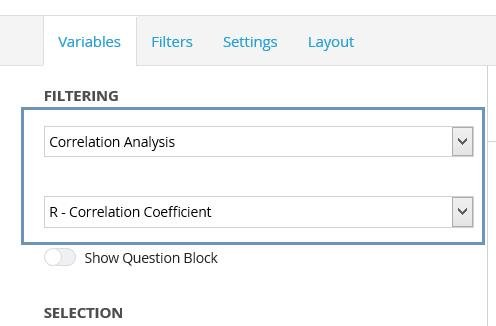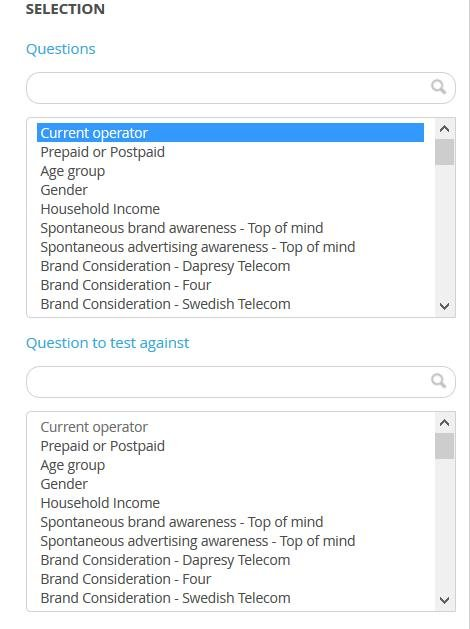The setup of the correlation is more or less identical to setting up a regular table or a chart with a mean or a percent calculation. The only difference is that two sets of variables need to be selected since the correlation test is performed between two variables.

A correlation test can be based on Single-choice Categorical or Numerical variables. A Categorical variable can be tested against a categorical or a numerical variable and vice versa. A correlation test cannot be applied to Multichoice Categorical variables due to the nature of the test.

The option for calculating the correlation is present in the ordinary calculation type drop-down list, as shown in the image below. A second drop-down list is used for selecting if the Correlation Coefficient ® or the Coefficient of Determination (R2) will be calculated. The image below shows the Correlation calculation options.When the type of correlation analyses option is selected (R or R2), the Administrator needs to select one or multiple variables to show in the chart/table as well as one variable to perform the test against. The image below shows the two selection boxes. The image below shows the variable selections when running a correlation analyses. The selected questions in the “Questions” list will be shown in the chart/table and be tested against the variable in the selection list named “Question to test against.”The other settings, such as selecting chart type, the number of decimals, filters, time periods, etc. are made in the same way as you would set up a chart or table showing a percentage calculation. The ordinary Analysis settings (significance tests, etc.) are hidden and unable to be used when the correlation calculation is used.